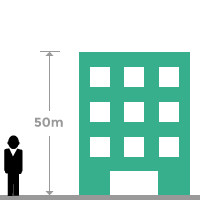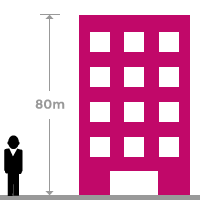# Rational numbersA number, which can be expressed as a ratio of two quantities is defined a Rational number.

Every rational number is usually formed by the division of two integers. Integers actually form rational numbers by working as antecedent and consequent of the ratio. The value of consequent should not be zero because it violates the rule of the division if zero is used as consequent of the ratio. Therefore, the ratio of an integer to a non-zero integer is known a rational number.

The comparison of the two integers returns a quotient as a result of division of the two integers. Similarly, a quotient can also be expressed as a ratio of two integers. The collection of rational numbers is denoted by the letter $Q$ which is first letter of the Quotient.

## How to use

The concept of rational numbers can be understood from an example. Consider two buildings. The heights of both buildings are $50$ and $80$ meters respectively.The heights $50$ and $80$ both are integers numerically. Now, calculate the ratio of height of the first building to height of the second building.

$Ratio=\frac{50}{80}$

$⇒Ratio=\frac{5}{8}$

It is a fraction but the antecedent $5$ and consequent $8$ both are integers in this ratio. It seems, the ratio of $5$ to $8$.

$⇒Ratio=\frac{5}{8}=0.625$

$⇒Ratio=\frac{5}{8}=0.625⇔0.625=\frac{5}{8}$

The quotient of ratio $5$ to $8$ is $0.625$ as per division. Similarly, the decimal number $0.625$ can also be expressed as a ratio of $5$ to $8$. Therefore, the ratio of $5$ to $8$ is $\frac{5}{8}$. It is called as a rational number.

Any two integers which are expressed as a ratio is called, a rational number. However, the integer zero $\left(0\right)$ should not be used in ratio as consequent. You understand the reason in the following example.

Consider integers $–4$ and $0$ and ratio of $–4$ to $0$ is written as follows.

$Ratio=\frac{–4}{0}$

The ratio is undefined because zero cannot divide the integer $–4$. Not only $–4$, it cannot divide any integer. For this reason, the integer zero cannot play role as consequent of ratio in forming the rational numbers.

### Representation

A rational number can be positive or negative fraction and also positive or negative integer. The collection of integers and fractions which can be expressed as a ratio of two integers and vice-versa, is known rational numbers. The set of Rational numbers is denoted by the letter $Q$.

$Q=\left\{\dots –2,–\frac{3}{2},–1,–\frac{1}{2},0,\frac{3}{4},1,\frac{7}{6},2,\dots \right\}$

Rational numbers is a collection of infinite numbers. So, the set of rational numbers is an example for infinite set.